# GSEB Solutions Class 7 Maths Chapter 10 Practical Geometry InText Questions

Gujarat Board GSEB Textbook Solutions Class 7 Maths Chapter 10 Practical Geometry InText Questions and Answers.

## Gujarat Board Textbook Solutions Class 7 Maths Chapter 10 Practical Geometry InText QuestionsThink, Discuss and Write (Page 195)

Question 1.
In the above construction (see Page 193 NCERT Textbook), can you draw any other line through A that would be also parallel to the line l?
Solution:
No, because through a given point only one line parallel to a line can be drawn.

Question 2.
Can you slightly modify the above construction (see Page 193 NCERT Textbook), to use the idea of equal corresponding angles instead of equal alternate angles?
Solution:
Yes.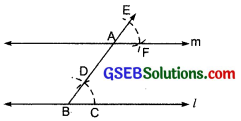In this case, with A as centre and radius equal to BC, draw an arc to cut BA (extended) at E.
Next, with centre as E and radius equal to CD mark a point F as shown in the figure.
Now join AF to draw a line m which is parallel to the given line ‘l’.
Here, the corresponding angles ∠CBE and ∠FAE are equal. Therefore, l || m.Think, Discuss and Write (Page 198)

Question 1.
A student attempted to draw a triangle whose rough figure is given here. He drew QR first. Then with as centre, he drew an arc of 3 cm and with R as centre, he drew an arc of 2 cm. But he could not get P. What is the reason? What property of triangle do you know in connection with this problem? Can such a triangle exist? (Remember the property of triangles ‘The sum of any two sides of a triangle is always greater than the third side;!)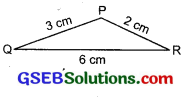Solution:
Such a triangle does not exist, because a triangle is possible only when the sum of the lengths of any two sides is greater than the third side. But here, 2 cm + 3 cm, i.e. 5 cm < 6 cm.

Think, Discuss and Write (Page 200)

Question 1.
In ∆ABC if AB = 3 cm, AC = 5 cm and m∠C = 30°. Can we draw this triangle?
Solution:
We may draw AC = 5 cm and ∠C = 30°. CA is one arm of ∠C. Point B should be lying on the other arm of ∠C. But we observe that point B cannot be located uniquely. So, the given data is not sufficient for construction of ∆ABC.

Think, Discuss and Write (Page 202)

Question 1.
In the above example (see Page 201, NCERT Textbook), length of a side and measures of two angles were given. Now study the following problem:
In ∆ABC, if AC = 7 cm, m∠A = 60° and m∠B = 50°, can you draw the triangle? (Angle- sum property of a triangle may help you!)
Solution:
We are given the line segment AC. ∠A is given but ∠C is not given. We can determine ∠C using angle-sum property.
∠C = 180° – (∠B + ∠A).
= 180° – (60° + 50°)
= 180° – 110° = 70°
Now, with the help of ∠C, we can construct the triangle.Miscellaneous Questions (Page 204)

Question 1.
Below are given the measures of certain sides and angles of triangles. Identify those which cannot be constructed and, say why you cannot construct them. Construct rest of the triangles.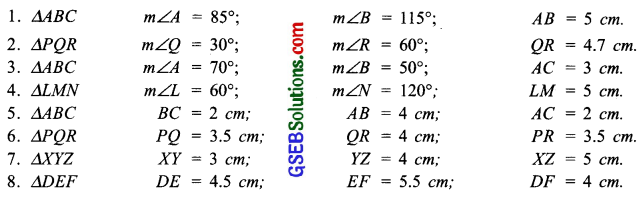Solution:
1. We have: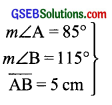Since for ∆ABC, ∠A + ∠B + ∠C = 180°
But here ∠A + ∠B = 85° + 115°
= 200° > 180°.
i. e. ∠A + ∠B + ∠C will be greater than 180°
Thus, ∆ABC cannot be constructed.

2. We have: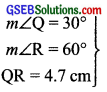Steps of construction:
I. Draw a line segment QR = 4.7 cm.
II. At Q, construct ∠RQX = 30°.
III. At R, construct ∠QRY = 60°.
IV. Let $$\overrightarrow{\mathrm{QX}}$$ and $$\overrightarrow{\mathrm{RY}}$$ intersect at R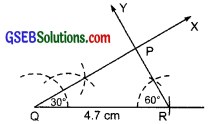Thus, ∆PQR is the required triangle.

3. We have: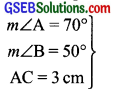Here, the side AC is given.
∴ To complete the triangle, we need m∠A and m∠C.
Since, m∠C = 180° – [∠A + ∠B]
= 180° – [70° + 50°]
= 180° – 120° = 60°
Steps of construction:
I. Draw a line segment AC = 3 cm.
II. At A, construct ∠CAX = 70°.
III. At C, construct ∠ACY = 60°
IV. Let the rays $$\overrightarrow{\mathrm{AX}}$$ and $$\overrightarrow{\mathrm{CY}}$$ meet at B.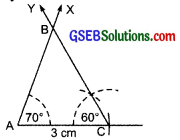∴ ∆ABC is the required triangle.

4. We have: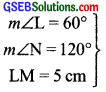Since, in ∆LMN,
∠L + ∠M +∠N = 180°
But here, ∠L + ∠N + ∠M
= 60° + 120° + ∠M = (180° + ∠M) > 180°.
∴ ∆LMN cannot be constructed.

5. We have:
$$\left.\begin{array}{l} \mathrm{BC}=2 \mathrm{~cm} \\ \mathrm{AB}=4 \mathrm{~cm} \\ \mathrm{AC}=2 \mathrm{~cm} \end{array}\right\}$$
We know that a triangle is possible only when the sum of lengths of any two sides is greater than the length of the third side.
Here BC + AC = 2 cm + 2 cm = 4 cm = AB
(∵ AB = 4 cm)
∴ ∆ABC is not possible.

6. We have:
$$\left.\begin{array}{l} \mathrm{PQ}=3.5 \mathrm{~cm} \\ \mathrm{QR}=4 \mathrm{~cm} \\ \mathrm{PR}=3.5 \mathrm{~cm} \end{array}\right\}$$
Steps of construction:
I. Draw a line PQ = 3.5 cm.
II. With centre P and radius 3.5 cm, draw an arc.
III. With centre Q and radius 4 cm, draw another arc, such that it cuts the previous arc at R.
IV. Join RP and RQ.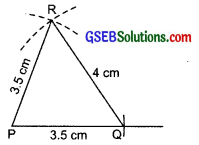Thus, ∆PQR is the required triangle.

7. We have:
$$\left.\begin{array}{l} \mathrm{XY}=3 \mathrm{~cm} \\ \mathrm{YZ}=4 \mathrm{~cm} \\ \mathrm{XZ}=5 \mathrm{~cm} \end{array}\right\}$$
Steps of construction:
I. Draw a line segment YZ = 4 cm.
II. With centre Y and radius 3 cm, draw an arc.
III. With centre Z and radius 5 cm, draw another arc which cuts the previous arc at X.
IV. Join XY and XZ.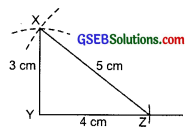Thus, ∆XYZ is the required triangle.

8. We have:
$$\left.\begin{array}{l} \mathrm{DE}=3 \mathrm{~cm} \\ \mathrm{EF}=4 \mathrm{~cm} \\ \mathrm{DF}=5 \mathrm{~cm} \end{array}\right\}$$
Steps of construction:
I. Draw a line segment EF = 5.5 cm
II. With centre E and radius 4.5 cm, draw an arc.
III. With centre F and radius 4 cm, draw another arc such that it intersects the previous arc at D.
IV. Join DE and DF.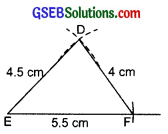Thus, ∆DEF is the required triangle.